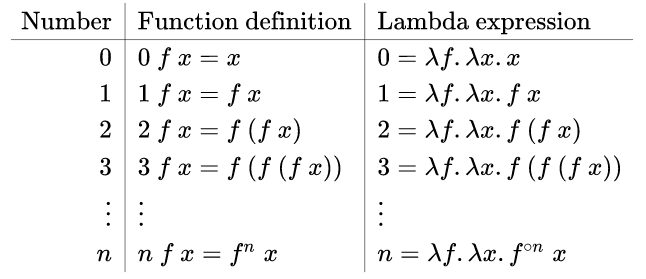# Jonathan Lam

Core Developer @ Hudson River Trading

## Blog

### SICP notes: Church numerals in Scheme

On 6/4/2021, 9:51:47 PM

These are notes spawned off of reading Structure and Interpretation of Computer Programs. I've been following along with the exercises, and my work can be found here. More of these posts may follow.

Here is a really interesting exercise:

In case representing pairs as procedures wasn't mind-boggling enough, consider that, in a language that can manipulate procedures, we can get by without numbers (at least insofar as nonnegative integers are concerned) by implementing 0 and the operation of adding 1 as

``````(define zero (lambda (f) (lambda (x) x)))]

(lambda (f) (lambda (x) (f ((n f) x)))))
``````

This representation is known as Church numerals, after its inventor, Alonzo Church, the logician who invented the calculus.

Define `one` and `two` directly (not in terms of `zero` and `add-1`). (Hint: Use substitution to evaluate `(add-1 zero)`). Give a direct definition of the addition procedure + (not in terms of repeated application of `add-1`).

Firstly, here are some function composition primitives (from section 1.3 exercises). Their functionality should be fairly clear by inspection, and their relevance will soon be apparent.

``````(define (identity x)
;; identity function
x)

(define (double f)
;; composes a function with itself
(lambda (x)
(f (f x))))

(define (compose f g)
;; returns the composition (f o g)
(lambda (x) (f (g x))))

(define (n-fold-compose f n)
;; generalized version of double: returns the function
;; obtained by composing f with itself n times. Assumes n>=0
(if [zero? n]
identity
(let iter ([n n])
(if [= n 1]
f
(compose f (iter (1- n)))))))
``````

Secondly, I like to annotate and namespace the examples from the book.

``````(define church-zero
;; Church numeral implementation of 0
(lambda (f)
(lambda (x) x)))

(define (church-1+ n)
;; Church numeral implementation of 1+
(lambda (f)
(lambda (x)
(f ((n f) x)))))
``````

`zero` is a procedure that always returns the identity function, so it is the same as writing:

``(define (church-zero f) identity)``

We immediately see that each "number" is a mapping into the space of procedures. We also see (from the definition of `church-1+`) that the domain of this mapping is necessarily also a procedure. I.e., each Church numeral is a mapping from functions to functions.1

The implementation of 1 in this function space is derived from the definitions of `church-zero` and `church-1+`.

``````(define (church-one f)
(lambda (x)
(f x)))
;;; simplify:
(define (church-one f) f)
;;; simplify:
(define church-one identity)
``````

We can apply the same method to derive `church-two`, the implementation of 2 in this representation:

``````(define church-two
(lambda (f)
(lambda (x)
(f (f x)))))
;;; simplify:
(define (church-two f)
(lambda (x)
(f (f x))))
;;; simplify:
(define church-two double)
``````

From this, the pattern starts to become clear: the Church numeral representation of a nonnegative integer n is the n-fold function composition operator.

The question asks us to write an explicit procedure for the sum of two Church numerals a and b. With the pattern above, we expect this to return the (a+b)-fold composition operator.

``````(define (church-add a b)
(lambda (f)
(lambda (x)
((a f) ((b f) x)))))
``````

Similarly, we can define a multiplication in this scheme, which returns the ab-fold composition operator.

``````(define (church-multiply a b)
;; multiply two Church numerals
(lambda (f)
(lambda (x)
((a (b f)) x))))
``````

At this point, we've defined a bijection between the nonnegative integers and the Church numerals.2

Then we can write functions that convert each nonnegative integer to its (unique) Church numeral representation and vice versa. For the forward direction, we perform n-fold composition on a function. For the backwards direction, we see how many times the Church numeral composes the `1+` function with itself, which very naturally reveals the corresponding integer.

``````(define (fx->church n)
;; nonnegative integer to corresponding Church numeral
(lambda (f)
(n-fold-compose f n)))

(define (church->fx n)
;; Church numeral to corresponding integer
((n 1+) 0))
``````

We can do a quick check to see that these work:

``````> (map church->fx (list church-zero church-one church-two))
(0 1 2)
> (church->fx (fx->church 512))
512
``````

As the name suggests, the Church numerals were invented by Alonzo Church, founder of lambda calculus and pioneer of computability theory, as a theoretical way to encode any data in the form of the primitives of lambda calculus: functions. Even without knowing lambda calculus notation, the Church numeral arithmetic in lambda calculus notation on the Wikipedia page for Church encoding are clearly equivalent to my Scheme definitions:Of course, Lisp was heavily influenced by lambda calculus, so this is hardly surprising. But I still find it very cool!

#### Footnotes

1. The terms mapping, procedure, and function are used interchangeably here. The first is used in math, the second in SICP, and the third in the general programming sense.

2. I recently finished my first Algebra class, so I want to elaborate a little. The nonnegative integers form a semiring (a ring without negatives, i.e., a rig or subring). We define the Church numeral n' to be the n-fold composition operator, and this automatically defines a bijective map g defined by g(n)=n'. Using the definitions of the Church addition and multiplication, and bootstrapping off of the properties of the integers, we can trivially prove two more facts: Church numerals also form a rig, and g is a subring homomorphism (a subring isomorphism.) I'm not sure if these facts are interesting though.